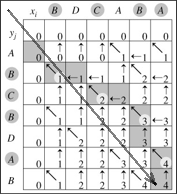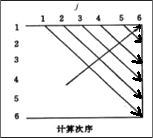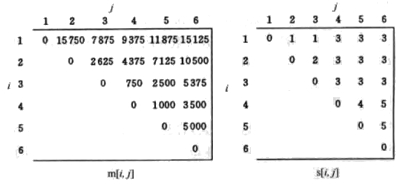# Dynamic Programming

## 1 动态规划简介

20世纪50年代初美国数学家Richard Bellman等人在研究多阶段决策过程(multistep decision process)的优化问题时，提出了著名的最优化原理(principle of optimality)，把多阶段过程转化为一系列单阶段问题，利用各阶段之间的关系，逐个求解，创立了解决这类过程优化问题的新方法——动态规划(Dynamic Programming)。

## 2 动态规划解决哪类问题

1. 最优子结构。问题具有最优子结构是指：一个问题的最优解中包含了子问题的最优解。
2. 重叠子问题。各个子问题中包含了公共的子子问题。动态规划能利用这种子问题的重叠性质，对每一个子问题只计算一次，然后将其计算结果保存下来，当再次需要计算已经计算过的子问题时，只是简单地查看一下以前保存的结果，从而获得较高的效率。

## 3 动态规则的解题步骤

(1)、寻找最优子结构。
(2)、利用子问题的最优解递归定义最优解的值（即先找到一个效率不高的递归解法）。
(3)、按自底向上的方式计算最优解的值。
(4)、从最后一步的最优值回溯，即可构造原始问题的最优解。

“最优解的值”是对“最优解”特征的部分描述。举例说明，最长公共子序列问题中，最长公共子序列的长度为“最优解的值”，而公共子序列具体包含哪此元素则是“最优解”。

## 4 实例：求最长公共子序列（Longest Common Subsequence, LCS）

### 4.1 问题分析

We solve the problem of finding the longest common subsequence of $$x=x_{1...m}$$ and $$y=y_{1...n}$$ by taking the best of the three possible cases:

1. The longest common subsequence of the strings $$x_{1...m-1}$$ and $$y_{1...n}$$
2. The longest common subsequence of the strings $$x_{1...m}$$ and $$y_{1...n-1}$$
3. If $$x_{m}$$ is the same as $$y_{n}$$, the longest common subsequence of the strings $$x_{1...m-1}$$ and $$y_{1...n-1}$$, followed by the common last character.

### 4.2 最长公共子序列之递归解法（自顶向下）

#include<stdio.h>
#include<string.h>

#define MAX(a,b) (((a)>(b))?(a):(b))

/* call it like this lcs_len(s, t, strlen(s)-1, strlen(t)-1) */
int lcs_len(char *s, char *t, int s_len, int t_len) {
if(s_len < 0 || t_len < 0) {
return 0;
}

if(s[s_len] == t[t_len]) {
return lcs_len(s, t, s_len-1, t_len-1)+1;
} else {
return MAX(lcs_len(s, t, s_len-1, t_len), lcs_len(s, t, s_len, t_len-1));
}
}

int main(void) {
char a[]="ABCBDAB";
char b[]="BDCABA";

printf("length: %d\n", lcs_len(a, b, strlen(a)-1, strlen(b)-1));    // 输出 length: 4
return 0;
}


### 4.3 最长公共子序列之动态规划解法（自底向上）

LCS-LENGTH(X, Y)
m ← length[X]
n ← length[Y]
for i ← 1 to m
do c[i, 0] ← 0
for j ← 0 to n
do c[0, j] ← 0
for i ← 1 to m
do for j ← 1 to n
do if xi = yj then
c[i, j] ← c[i - 1, j - 1] + 1
b[i, j] ← "↖"
else if c[i - 1, j] ≥ c[i, j - 1] then
c[i, j] ← c[i - 1, j]
b[i, j] ← "↑"
else c[i, j] ← c[i, j - 1]
b[i, j] ← "←"
return c and b


PRINT-LCS(b, X, i, j)
if i = 0 or j = 0
then return
if b[i, j] = "↖"
then PRINT-LCS(b, X, i - 1, j - 1)
print xi
else if b[i, j] = "↑"
then PRINT-LCS(b, X, i - 1, j)
else PRINT-LCS(b, X, i, j - 1)Figure 1: 动态规划实例：求解LCS问题

1 中，从左上角到右下角的大箭头表示 $$c[i][j]$$ 和 $$b[i][j]$$ 中元素的计算顺序。

#### 4.3.1 动态规划算法实现

// file LCS_DP.cpp 动态规划求解LCS问题。
#include<string.h>
#include<stdio.h>

enum Directions {kInit = 0, kLeft, kUp, kLeftUp};  // directions of LCS generation

void LCS_Print(int **LCS_direction,const char* pStr1, size_t row, size_t col);

// Get the length of two strings' LCSs, and print one of the LCSs
// Input: pStr1         - the first string
//        length1       - strlen(pStr1)
//        pStr2         - the second string
//        length2       - strlen(pStr2)
// Output: the length of two strings' LCSs
int LCS(const char* pStr1, size_t length1, const char* pStr2, size_t length2) {
size_t i, j, lcs_length;

// initiate the length matrix
int **LCS_length;
LCS_length = new int*[length1];
for(i = 0; i < length1; ++ i)
LCS_length[i] = new int[length2];

for(i = 0; i < length1; ++ i)
for(j = 0; j < length2; ++ j)
LCS_length[i][j] = 0;

// initiate the direction matrix
int **LCS_direction;
LCS_direction = new int*[length1];
for( i = 0; i < length1; ++ i)
LCS_direction[i] = new int[length2];

for(i = 0; i < length1; ++ i)
for(j = 0; j < length2; ++ j)
LCS_direction[i][j] = kInit;

//计算LCS_length和LCS_direction。
for(i = 0; i < length1; ++ i) {
for(j = 0; j < length2; ++ j) {
if(i == 0 || j == 0) { //这是个边界的情况，应单独拿出来，否则i-1等会出界。
if(pStr1[i] == pStr2[j]) { //这个情况也得考虑。
LCS_length[i][j] = 1;
LCS_direction[i][j] = kLeftUp;
}
else
LCS_length[i][j] = 0; //可以去掉，因为前面已经全部初始为0了。
}
// a char of LCS is found,
// it comes from the left up entry in the direction matrix
else if(pStr1[i] == pStr2[j]) {
LCS_length[i][j] = LCS_length[i - 1][j - 1] + 1;
LCS_direction[i][j] = kLeftUp;
}
// it comes from the up entry in the direction matrix
else if(LCS_length[i - 1][j] > LCS_length[i][j - 1]) {
LCS_length[i][j] = LCS_length[i - 1][j];
LCS_direction[i][j] = kUp;
}
// it comes from the left entry in the direction matrix
else { //最后剩下情况是LCS_length[i - 1][j] <= LCS_length[i][j - 1]
LCS_length[i][j] = LCS_length[i][j - 1];
LCS_direction[i][j] = kLeft;
}
}
}
LCS_Print(LCS_direction, pStr1, length1 - 1, length2 - 1);   //调用下面的LCS_Pring打印出所求子串。

lcs_length = LCS_length[length1 - 1][length2 - 1];
//释放二维数组空间。
for(i=0; i < length1; i++)
delete[] LCS_length[i];
delete[] LCS_length;

for(i=0; i < length1; i++)
delete[] LCS_direction[i];
delete[] LCS_direction;

return lcs_length;    //返回长度。
}

// Print a LCS for two strings
// Input: LCS_direction - a 2d matrix which records the direction of
//                        LCS generation
//        pStr1         - the first string
//        row           - the row index in the matrix LCS_direction
//        col           - the column index in the matrix LCS_direction
void LCS_Print(int **LCS_direction,
const char* pStr1,
size_t row, size_t col) {
if(pStr1 == NULL)
return;

// kLeftUp implies a char in the LCS is found
if(LCS_direction[row][col] == kLeftUp) {
if(row > 0 && col > 0)
LCS_Print(LCS_direction, pStr1, row - 1, col - 1);
// print the char
printf("%c", pStr1[row]);
}
else if(LCS_direction[row][col] == kLeft) {
// move to the left entry in the direction matrix
if(col > 0)
LCS_Print(LCS_direction, pStr1, row, col - 1);
}
else if(LCS_direction[row][col] == kUp) {
// move to the up entry in the direction matrix
if(row > 0)
LCS_Print(LCS_direction, pStr1, row - 1, col);
}
}

int main() {
char a1[]="BDCABA";
char b1[]="ABCBDAB";

printf(" length %d\n", LCS(a1, strlen(a1), b1, strlen(b1)));   // 输出 BCBA length 4

char a2[] = "GCGCAATG";
char b2[] = "GCCCTAGCG";

printf(" length %d\n", LCS(a2, strlen(a2), b2, strlen(b2)));   // 输出 GCCTG length 5

return 0;
}


## 5 实例：求最大子序和

### 5.2 动态归划算法实现

//用动态规划算法解决最大子序和问题。
#include<iostream>

using std::cout;
using std::endl;

//返回值为子数组的最大和。
//A[]为数组，N为数组元素个数，start和end分别返回子数组开始、结束位置。
int max_sub_array(const int A[], int N, int& start, int& end) {
int b = A;
int sum = A;
int start_tmp = 0;
int end_tmp = 0;    //start_tmp和end_tmp用来辅助定位start、end。
for (int j = 1; j < N; j++) {
if (b > 0) {
b = b + A[j];
end_tmp = j;
} else {
b = A[j];
start_tmp = end_tmp = j;
}
if (sum < b) {
sum = b;
start = start_tmp;
end = end_tmp;
}
}
return sum;
}

int main() {
int A[] = { -2, 11, -4, 13, -5, -2 };
int start, end;
cout << max_sub_array(A, 6, start, end) << endl;
cout << "start: A" << start << endl;  // 这个例子会输出 start: A1
cout << "end: A" << end << endl;      // 这个例子会输出 end: A3
}


### 5.3 扩展问题：求最大子矩阵

 0 -2 -7  0
9  2 -6  2
-4  1 -4  1
-1  8  0 -2


 9 2
-4 1
-1 8


POJ（北京大学程序在线评测系统）上有这个题：http://poj.org/problem?id=1050

## 6 实例：求编辑距离（Edit Distance）

(1) 插入一个字符；
(2) 删除一个字符；
(3) 替换一个字符。

Levenshtein distance Wikipedia: https://en.wikipedia.org/wiki/Levenshtein_distance

### 6.1 问题分析

(1) 如果a替换为b时，可使A变为B，则 ed(i,j) = ed(i-1,j-1) + 1；
(2) 如果在a后面插入字符d，可使A变为B，则 ed(i,j) = ed(i,j-1) + 1；
(3) 如果将a删除，可使A变为B，则 ed(i,j) = ed(i-1,j) + 1。

$ed(i,j) = \begin{cases} i & \text{if} \; j = 0 \\ j & \text{if} \; i = 0 \\ ed(i-1,j-1) & \text{if} \; i,j > 0; a_i = b_j \\ \min\{ed(i-1,j-1), ed(i,j-1), ed(i-1,j)\} + 1 & \text{if} \; i,j > 0; a_i \ne b_j \\ \end{cases}$

### 6.2 递归解法

// len_s and len_t are the number of characters in string s and t respectively
int EditDistance(char *s, int len_s, char *t, int len_t)
{
int cost;

/* base case: empty strings */
if (len_s == 0) return len_t;
if (len_t == 0) return len_s;

/* test if last characters of the strings match */
if (s[len_s-1] == t[len_t-1]) {
return EditDistance(s, len_s - 1, t, len_t - 1);
} else {
int minimum = min(EditDistance(s, len_s - 1, t, len_t), EditDistance(s, len_s, t, len_t - 1));
return min(minimum, EditDistance(s, len_s - 1, t, len_t - 1)) + 1;
}
}


### 6.3 动态规划解法

#include <string>
#include <iostream>
using namespace std;

int EditDistance(const string & word1, const string & word2) {
int n = word1.length();
int m = word2.length();

int dp[n+1][m+1];

for(size_t i=0; i<=n; i++){
dp[i] = i;
}
for(size_t j=0; j<=m; j++){
dp[j] = j;
}

for(size_t i=1; i<=n; i++){
for(size_t j=1; j<=m; j++){
if(word1[i-1] == word2[j-1]){
dp[i][j] = dp[i-1][j-1];
}else{
dp[i][j] = min(dp[i-1][j-1], min(dp[i-1][j],dp[i][j-1])) + 1;
}
}
}

return dp[n][m];
}

int main() {
string A = "GGATCGA";
string B = "GAATTCAGTTA";
cout << EditDistance(A,B) << endl;  // 输出 5
}


## 7 实例：求矩阵链乘法

$\begin{gathered} (A_1(A_2(A_3A_4))) \\ (A_1((A_2A_3)A_4)) \\ ((A_1A_2)(A_3A_4)) \\ ((A_1(A_2A_3))A_4) \\ (((A_1A_2)A_3)A_4) \\ \end{gathered}$

### 7.1 问题分析

(1)、寻找最优子结构。

(2)、建立递归关系。

$m[i][j] = m[i][k] + m[k+1][j] + p_{i-1}p_kp_j$

$m[i][j] = \begin{cases} 0 & \text{if} \; i=j \\ \min_{i \le k \lt j} \{m[i][k] + m[k+1][j] + p_{i-1}p_kp_j \} & \text{if} \; i< j \end{cases}$

### 7.2 动态规划算法实现

//矩阵链乘法的动态规划算法实现。
//m[i][j]和s[i][j]的下标都从1开始，这是为了和前面的描述尽量一致。
//代码参考了《计算机算法设计与分析(第3版, 王晓东编)》3.1节。
#include<iostream>
#include<iomanip>    //使用setw()时需要
using std::cout;
using std::endl;
using std::setw;

#define N 6  //矩阵个数

//p为矩阵维数数组，n为矩阵个数。
void MatrixChain(int *p, int n, int m[][N + 1], int s[][N + 1]) {
for (int i = 1; i <= n; i++) {
m[i][i] = 0; //i=j时，m[i][j]为0。
}
for (int l = 2; l <= n; l++) {  //子序列长度由2到n递增
for (int i = 1; i <= n - l + 1; i++) {  //注意i的上限
int j = i + l - 1;
m[i][j] = m[i + 1][j] + p[i - 1] * p[i] * p[j];
s[i][j] = i;
for (int k = i + 1; k < j; k++) {
if (m[i][j] > m[i][k] + m[k + 1][j] + p[i - 1] * p[k] * p[j]) { //根据递归表达式计算m[i][j]
m[i][j] = m[i][k] + m[k + 1][j] + p[i - 1] * p[k] * p[j];
s[i][j] = k;  //记录s[i][j]
}
}
}
}
}

//递归打印加上括号的矩阵链。
//s为保存着辅助信息的数组。i,j为矩阵链范围。
void Print_parens(int s[][N + 1], int i, int j) {  //递归地构造最优解
int k = s[i][j];
if (i != j) {
if (!(i == 1 && j == N)) {
cout << "(";
}
Print_parens(s, i, k);
Print_parens(s, k + 1, j);
if (!(i == 1 && j == N)) {
cout << ")";
}
} else {
cout << "A" << i;
}
}

int main() {
int p[N + 1] = { 30, 35, 15, 5, 10, 20, 25 };
int m[N + 1][N + 1] = { 0 };  //不初赋值不会影响结果，但m的“下三角数组”会为随机数。
int s[N + 1][N + 1] = { 0 };  //不初赋值不会影响结果，但s的“下三角数组”会为随机数。

MatrixChain(p, N, m, s);
Print_parens(s, 1, N);

//打印m数组和s数组。
cout << endl << endl;
cout << "m=" << endl;
for (int i = 1; i <= N; i++) { //由于前面约定数组从1开始。
for (int j = 1; j <= N; j++) {
cout << setw(8) << m[i][j] << " ";
}
cout << endl;
}
cout << endl;
cout << "s=" << endl;
for (int i = 1; i <= N; i++) {
for (int j = 1; j <= N; j++) {
cout << setw(8) << s[i][j] << " ";
}
cout << endl;
}
return 0;
}


(A1(A2A3))((A4A5)A6)

m=
0    15750     7875     9375    11875    15125
0        0     2625     4375     7125    10500
0        0        0      750     2500     5375
0        0        0        0     1000     3500
0        0        0        0        0     5000
0        0        0        0        0        0

s=
0        1        1        3        3        3
0        0        2        3        3        3
0        0        0        3        3        3
0        0        0        0        4        5
0        0        0        0        0        5
0        0        0        0        0        0


#### 7.2.1 程序相关说明

Table 1: 求矩阵链乘法测试程序所用数据
$$A_1$$ $$A_2$$ $$A_3$$ $$A_4$$ $$A_5$$ $$A_6$$
30×35 35×15 15×5 5×10 10×20 20×25Figure 2: 求矩阵链乘法程序中数组m和s的计算次序Figure 3: 求矩阵链乘法程序中数组m和s的最终结果

Created: <2011-08-27 Sat 00:00>

Last updated: <2017-12-17 Sun 00:03>

Creator: Emacs 25.3.1 (Org mode 9.1.4)